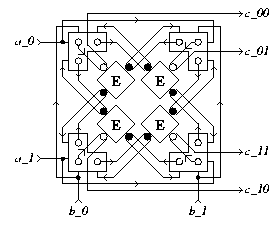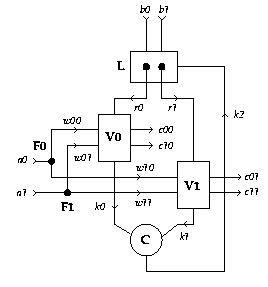EDIS: Guide | FAQ | New | Search | Bibliography | Index | Feedback

# Decision-Wait

## Specifications

### Informal

A Decision-Wait has four input terminals (a_0, a_1, b_0, and b_1), and four output terminals (c_00, c_01, c_10, and c_11). It waits for a signal on one of the a inputs and a signal on one of the b inputs. The environment has to guarantee mutual exclusion on the a-inputs and also the b-inputs. Having received input signals on a_i (i=0 or i=1) and b_j (j=0 or j=1), it produces a signal on output terminal c_ij.

Rephrasing: A Decision-Wait receives two bits of data in a Double-Rail code via the a and b inputs and converts them to a four-wire One-Hot code via the c outputs. Phrased yet differently: It awaits two binary decisions and reports the combination.

### XDI

Schematic diagram for a Decision-Wait DW(a_0, a_1, b_0, b_1; c_00, c_01, c_10, c_11):[Zoom|FIG]

XDI state graph for a Decision-Wait:[Zoom|FIG]

Specification in XDI model.

### Verdect

Specification in Verdect:

```
define JOIN2X2( a0?, a1?, b0?, b1?, c00!, c01!, c10!, c11! ) =
pref *[ (a0? || b0?); c00!
| (a0? || b1?); c01!
| (a1? || b0?); c10!
| (a1? || b1?); c11!
]
end
```

### DI Algebra

Specification in DI Algebra.

## Properties

XDI Report.

The Decision-Wait is very symmetric, having 8 automorphisms. The roles of subscripts 0 and 1 can be interchanged, independently on both a and on b, and also the roles of a and b can be interchanged, provided that the c outputs are permuted accordingly:
DW(a_0, a_1, b_0, b_1; c_00, c_01, c_10, c_11) =
DW(a_1, a_0, b_0, b_1; c_10, c_11, c_00, c_01) =
DW(a_0, a_1, b_1, b_0; c_01, c_00, c_11, c_10) =
DW(a_1, a_0, b_1, b_0; c_11, c_10, c_01, c_00) =
DW(b_0, b_1, a_0, a_1; c_00, c_10, c_01, c_11) =
DW(b_1, b_0, a_0, a_1; c_01, c_11, c_00, c_10) =
DW(b_0, b_1, a_1, a_0; c_10, c_00, c_11, c_01) =
DW(b_1, b_0, a_1, a_0; c_11, c_01, c_10, c_00)

The Decision-Wait satisfies Rules Y' and Z^out, but not Z^in (choice between a inputs, and also between b inputs).

## Implementations

### DI Decompositions

1. A Decision-Wait can be implemented using four Partial Decision-Waits, four E-Elements, and four Forks (not equ because the implementation accepts a_0a_1):[Zoom|FIG]
2. A Decision-Wait can be implemented using two Non-Receptive 1-Bit Variables, a Latch, two Forks and a Join (equ):[Zoom|FIG]

The Ludwig verification script of this implementation.

### Using Boolean Gates

No information available

### Using Transistors

No information available

## Generalizations

The Decision-Wait can be generalized in several ways. It can be generalized to an mxn-Decision-Wait, which awaits two choices, one between m inputs and the other between n inputs, and reports the combination as a choice among mn outputs. A Decision-Wait is a 2x2-Decision-Wait.

It can be further generalized to an n-dimensional v-decision-wait with #v=n>0, which awaits n choices, choice i being among v.i inputs, and reports the combination as a choice between (PROD i: 0 <= i < n: v.i) outputs. A Decision-Wait is a 2-dimensional (2,2)-Decision-Wait.

## Miscellaneous

In [Dickson74, p. 48], the Decision-Wait and its generalizations are referred to as Decision Rendezvous.

In [Molnar74, Part 1, Volume III, p. 13], the Decision-Wait and its generalizations are referred to as D Element.

In terms of [Ebergen89, p. 88], the Decision-Wait and its two-dimensional generalizations are CAL components. In [Ebergen89, pp. 95-99], decompositions of CAL components in terms of ... are presented.

Conjecture: A Decision-Wait cannot be implemented with only Forks, Joins, Merges, Latches (or even Sequencers), and I-Wires.

## References

[Dickson74, p. 48]
[Ebergen89, p. 88]
[Ebergen89, pp. 95-99]
[Molnar74, Part 1, Volume III, p. 13]